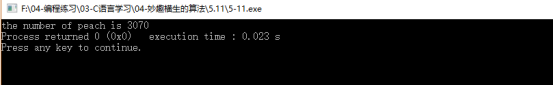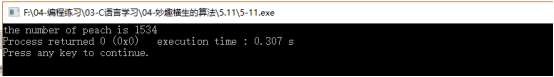# 2. 题目分析

【问题】如何确保桃子的一半一定是一个偶数呢？

【注意】i++还是++i

# 3. 代码尝试

## 【版本1】

#include "stdio.h"

int main(){

int num=1;

int i=1;

while(i<=10){

num = (num+1)*2;

//++i;

i++;

}

printf("the number of peach is %d",num);

}## 【最终版本】

#include "stdio.h"

int main(){

int num=1,i;

for(i=9;i>0;i--){

num = (num+1)*2;

}

printf("the number of peach is %d",num);

}

#include "stdio.h"

int main(){

int num=1;

int i=1;

while(i<=9){

num = (num+1)*2;

//++i;

i++;

}

printf("the number of peach is %d",num);

}0条评论